# Weyl tensor

(diff) ← Older revision | Latest revision (diff) | Newer revision → (diff)

In Riemannian geometry one has a manifoldof dimensionwhich admits a metric tensorwhose signature is arbitrary. Letbe the unique Levi-Civita connection onarising fromand letbe the associated curvature tensor with components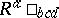. Of importance in Riemannian geometry is the idea of a conformal change of metric, that is, the replacement of the metricby the metric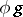whereis a nowhere-zero real-valued function on. The metricsand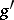are then said to be conformally related (oris said to be "conformal" to). One now asks for the existence of a tensor onwhich is constructed from the original metric onand which would be unchanged if it were to be replaced with another metric conformally related to it. (It is noted here that the curvature tensor would only be unaffected by such a change, in general, if the functionwere constant.) The answer was provided mainly by H. Weyl [a1], but with important contributions from J.A. Schouten [a2] (see also [a3]). For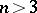, Weyl constructed the tensor(now called the Weyl tensor) with components given by(a1)where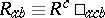are the Ricci tensor components,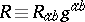is the Ricci scalar and square brackets denote the usual skew-symmetrization of indices. If this tensor is written out in terms of the metricand its first- and second-order derivatives, it can then be shown to be unchanged ifis replaced by the metric. (It should be noted that this is not true of the tensor with components, which would be scaled by a factoron exchangingfor.) Ifis a flat metric (so that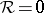), then the Weyl tensor constructed from(and from) is zero on. Conversely, ifgives rise, from (a1), to a zero Weyl tensor on, then for eachinthere are a neighbourhoodofin, a real-valued functiononand a flat metriconsuch thaton(i.e.is locally conformal to a flat metric on). Whenon, the latter is called conformally flat.

If, it can be shown from (a1) that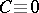on. Since not every metric on such a manifold is locally conformally related to a flat metric, the tensoris no longer appropriate. The situation was resolved by Schouten [a2] when he found that the tensor given in components by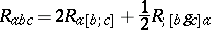(a2)

(using a semi-colon to denote a covariant derivative with respect to the Levi-Civita connection arising from the metric) played exactly the same role in dimensionas didfor. If, every metric onis locally conformally related to a flat metric [a3].

The tensorhas all the usual algebraic symmetries of the curvature tensor, together with the extra relation. If the Ricci tensor is zero on, the Weyl tensor and the curvature tensor are equal on. The tensor introduced in (a2) by Schouten possesses the algebraic identities(a3)

It is interesting to ask if two metrics on() having the same Weyl tensor as in (a1) are necessarily (locally) conformally related. The answer is clearly no if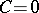. Ifis not zero on, the answer is still no, a counter-example (at least) being available for a space-time manifold (i.e. a-dimensional manifold admitting a metric with Lorentz signature).

The Weyl tensor finds many uses in differential geometry and also in Einstein's general relativity theory. In the latter it has important physical interpretations and its algebraic classification is the famous Petrov classification of gravitational fields [a4]

How to Cite This Entry:
Weyl tensor. Encyclopedia of Mathematics. URL: http://encyclopediaofmath.org/index.php?title=Weyl_tensor&oldid=13123
This article was adapted from an original article by G.S. Hall (originator), which appeared in Encyclopedia of Mathematics - ISBN 1402006098. See original article